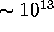Next: Young Energetic pulsars Up: A Tutorial on Radio Previous: The population of millisecond

# Assignment 2

1. Assuming a thin-screen model, calculate the apparent angular diameter of the Crab pulsar at 600 MHz. Assume that the apparent angular diameter = 100mas at 100 MHz.
2. Calculate the apparent angular diameter at 600 MHz if the screen is located at 1% of the distance from the pulsar to the Earth. Hint: write down a formula for the geometry in question 1 and the make a substitution for the distance from the pulsar to the screen.
3. Calculate the parallax's for pulsars with distances of 100, 500, 1000, 5000, 10000 parsecs.
4. Would it be possible to measure these parallax's using MERLIN? Why? If so suggest an observational strategy ie how many epochs of observations and how you would space them.
5. Compare the characteristic age of the Crab pulsar with the time since Chinese astronomers observed the supernova that formed the pulsar. Try using the Princeton pulsar catalog service on the WWW to get the parameters you need.
6. Calculate the birth period of a pulsar which has the following parameters: P = 1.87 ms, n = 3, t = 5 billion years and. Why is this number interesting?

Jon Bell
Thu Dec 19 15:15:11 GMT 1996